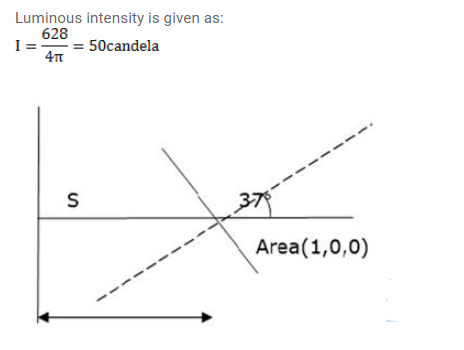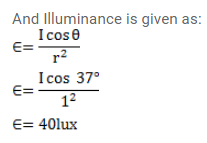# A point source emitting 628 lumen of luminous flux uniformly

Question:

A point source emitting 628 lumen of luminous flux uniformly in all direction is placed at the origin. Calculate the illuminance on a small area placed at $(1.0 \mathrm{~m}, 0,0)$ in such a way that the normal to the area makes an angle of $37^{\circ}$ with the $X$-axis.

Solution: